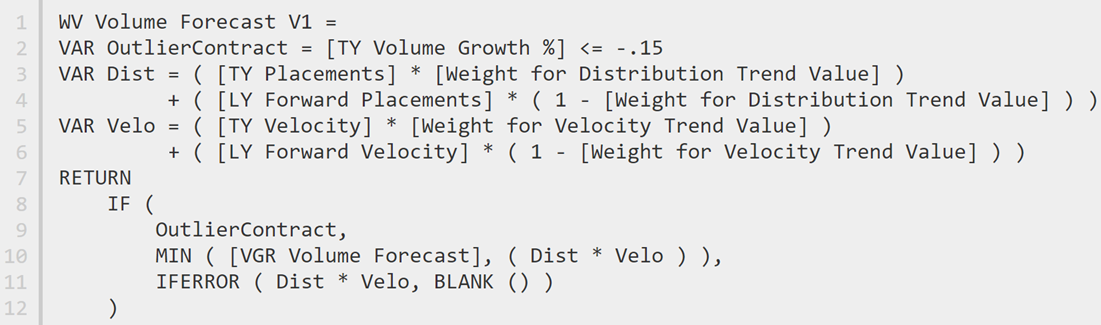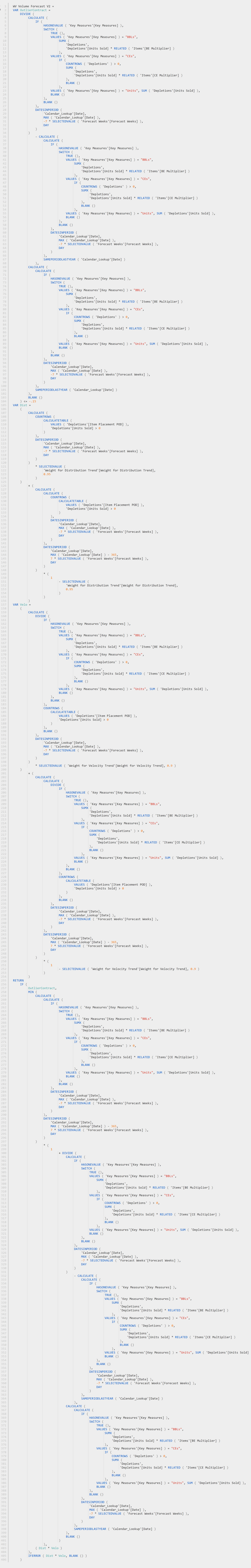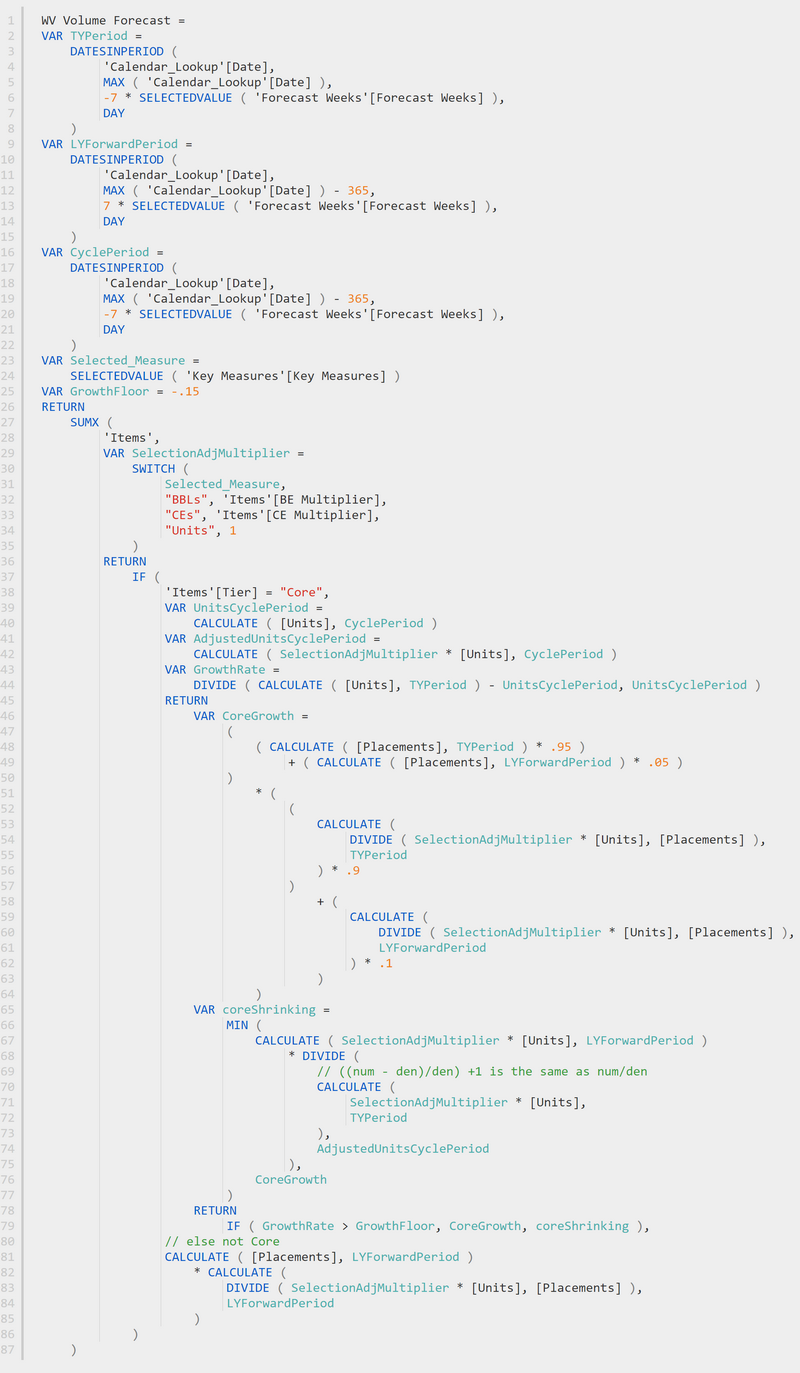Exploding Measures using Tabular Editor 3 - Excelerator BI

# Exploding Measures using Tabular Editor 3

What?! Exploding Measures I hear you say. Exploding, expanding, use which ever word you like; what I mean is when you want to remove any refence to a measure INSIDE another measure.

## Example

Consider the 3 measures below.

```Total Sales = SUM(Sales[ExtendedAmount])
Total All Product Sales = CALCULATE([Total Sales],ALL(Products))
% Of All Product Sales = DIVIDE([Total Sales],[Total All Product Sales])
```

The final measure, % Of All Product Sales refers to 2 interim measures. There are 2 references to other measures inside the third measure. This is very common practice, and there are many benefits of writing your DAX this way.

## The Benefits

• You get to write the long form formula once, and reuse it many times.
• This is shorter and faster
• If you need to change the base formula, you only have to change it in one location
• The logic of the formula is easier to read and understand because the measure names are descriptive whereas the underlying formulas may require deep analysis to work out what they do.
• You can break the DAX problem into logical components and solve one part of the overall calculation problem before moving on to the next.

## The Problem

OK, so what’s the problem? The problem is that sometimes you get such a deep, nested path of measures that the underlying formula is more complex than it needs to be, and it is virtually impossible to debug. If you simply explode/expand a final measure into the full, underlying DAX code, it makes it much easier to see what is going on. Returning to the DAX example above, this is what the third measure actually looks like under the hood, removing all references to the dependent measures

```% Of All Product Sales =
DIVIDE (
SUM ( Sales[ExtendedAmount] ),
CALCULATE (
SUM ( Sales[ExtendedAmount] ),
ALL ( Products ) )
)```

In this simple example above, I am not saying that the fully exploded/expanded DAX is more efficient; this is just an illustration. But the following example IS a problem

## Original DAX Formula

Below is an actual DAX formula that was performing poorly for my customer. It was 12 lines of code.## Fully Exploded Formula

The fully exploded version of this exact same formula (see below) is 406 lines of code. See the problem?  I have a video down below that shows how to quickly turn the Original DAX version into the Fully Exploded Version using Tabular Editor 3.## So which Is Faster?

Well in this case, they are identical in speed, because the fully exploded formula is identical in operation to the original formula. Actually, that may not be strictly true due to every measure having an implicit CALCULATE(), but that is a much bigger conversation beyond the scope of this article. What is important in this instance is to

• Understand there is a problem.
• Know how to explode the measure into its full component DAX (see video below)
• Once exploded, restructure and rewrite the DAX so that it is more efficient.

Here is my final version in case you are interested. The original version took around 45 seconds to run. This final version runs in 66 milliseconds.  To improve the DAX, I did the following:

• Analysed what the DAX was doing
• Found the repeating patterns and identified which could be evaluated once in a single pass, bringing these into variables at the start of the measure
• Worked out how the iteration through the SUMX worked, and simplified it so it did it once only.## Here is the Video of how to Explode your Measures

### 3 thoughts on “Exploding Measures using Tabular Editor 3”

1.Interesting
Could you explain, or show, how you found and fixed the problems in this measure once it was exploded?

1.That’s a bigger topic :-). No promises, but I will take a look and see.

2.Great article Matt, thanks a lot!!

Scroll to Top